# Experimental investigation of turbulent flow convection heat transfer of MgO/water nanofluid at low concentrations – Prediction of aggregation effect of nanoparticles

Experimental investigation of turbulent flow convection heat transfer of MgO/water nanofluid at low concentrations – Prediction of aggregation effect of nanoparticles

Department of Chemical Engineering, University of Isfahan, Isfahan, Iran

Department of Petroleum Engineering, Petroleum University of Technology, Ahwaz, Iran

Corresponding Author Email:
asolaimany@eng.ui.ac.ir
Page:
755-764
|
DOI:
https://doi.org/10.18280/ijht.350409
|
Accepted:
|
Published:
31 December 2019
| Citation

OPEN ACCESS

Abstract:

The extent of increase in the convection heat transfer of MgO/water nanofluid was investigated at low concentrations within the range of 0.02 to 0.12 % vol, under turbulent flow and within the Reynolds number range of 11,000 to 49,000. It was found that at about 12 %, the heat transfer coefficient was increased compared with the base fluid, where on average, around 6 % increase was observed within the entire concentration range and the investigated Reynolds number. The aggregate effect of particles was examined in predicting the models for the determination of the physical properties of thermal conductivity and viscosity. It was observed that fractal models enjoy a greater accuracy when compared with other models. In addition, a model was proposed to predict the local heat transfer coefficient, in which the aggregate effect of nanoparticles was also investigated. It was observed that the relative average deviation of the proposed model is around 2.5 %, when compared with experimental values.

Keywords:

aggregate, low concentration, mgo/water nanofluid, physical properties

1. Introduction

In recent decades, the issue of saving energy has become a mainstream interest for researchers considering economic and environmental aspects. The new technology of applying nanofluid compounds in heat transfer instruments has culminated in development of a new branch of research in the area of heat transfer. The common objective of all researchers who are active in the area of application of nanofluids in heat transfer is to improve heat transfer, efficiency, and enhance the performance of heat devices. These results in both energy-saving and smallness of devices, thereby decreasing the cost of materials and development of devices. Application of nanofluids as a heat exchanger fluid in heat exchange devices has attracted lot of attention over the last two decades.

Choi et al  first propounded application of particles suspended in base fluid in order to improve the rate of heat transfer. Thereafter, many attempts have been carried out to better understand the changes in the convection heat transfer coefficient in heat exchangers. Research results have revealed that the heat transfer coefficient of nanofluids at low volumetric concentrations is greater than in base fluid, while the changes in friction coefficient and viscosity of the nanofluid are relatively low and trivial. Hence, the application of low concentrations of nanoparticles in the base fluid became the agenda of researchers. On the other hand, a number of researchers began to determine the equations for predicting convection heat transfer coefficient [2-11].

The important point in predicting the equations of convection heat transfer coefficient was selecting the suitable relations for determining the physical properties of nanofluids especially thermal conductivity and viscosity. The greater the accuracy of relations in predicting the physical properties of nanofluid, the lesser the error in the values obtained from the equations of convection heat transfer coefficient.

Duangthongsuk & Wongwise  determined common equations utilized in a large number of researches to determine the physical properties of nanofluids. Motevasel et al  demonstrated that with the increase in the concentration, the values calculated for conductivity grew in difference with the common models. Hence, the variety in application of these equations at high concentrations will result in difference between the values of calculated heat transfer coefficient. However, at low concentrations, the difference between the calculated values is trivial and the responses are almost similar. Moreover, in any case, even at low concentrations, the values calculated by conventional models are different with real and experimental values. Xuan and Li  stated particles aggregation as one of the important factors in the difference between the real and calculated values.

In calculating the physical properties of nanofluids, conductivity and viscosity alongside the prediction of heat transfer equations, researchers choose and investigate models assuming that the mean diameter of particles in solution or particle state same as in powder state, although due to the presence of Van der Waals force, the particles tend to aggregate  and this effect has not been examined in equations utilized in determining physical properties as well as in heat transfer coefficient equations.

The aim of this research is to examine the effect of nanoparticles aggregation and select a suitable equation for determining physical properties by considering this effect. In addition, in heat transfer models, nanoparticles aggregation can also influence some transfer mechanisms investigated in this research and consider the model presented for determining the heat transfer coefficient. Therefore, overall in the predicted heat transfer model, the effect of aggregation on conductivity and viscosity, important physical properties of nanofluids, has been investigated in predicting heat transfer coefficient. In carrying out this research, a set of heat transfer devices, operating in the form of a closed cycle, was utilized for measuring the convection heat transfer coefficient experimentally under constant heat flux conditions. Distilled water was utilized as the base fluid and magnesium oxide nanoparticles, which have been understudied so far [16-18], were used for the preparation of nanofluid within the volume concentration of 0.02 to 0.12 % and for five different concentrations along with 16 different rates within the turbulent flow range and approximate Reynolds number in the range of 11,000-49,000. To experimentally measure the physical properties of the nanofluid, appropriate measurement devices were utilized. To determine the bulking degree alongside the size and distribution of aggregates, dynamic light scattering (DLS) device was used.

2. Experimental Framework

2.1. Materials

The nanoparticles utilized in this research were magnesium oxide made by US Nano Co. The specifications of the mentioned nanoparticles include: mean diameter of particles of 20 nm, thermal conductivity of 48 W.m-1.K-1, heat capacity of 880 J.kg-1.K-1, and density of 3580 kg.m-3.

2.2. Sample preparation

The concentrations utilized in this research ranged from 0.02 to 0.12 % volumetric concentrations. To prepare the nanofluid, distilled water was utilized. After weighing the required samples with a certain volume concentration by a balance (ABI 100_5m_kern Corp.) with maximum error of 0.5 %, it was mixed in a certain volume of distilled water by a magnetic stirrer for 1 h. Thereafter, it was subjected to an ultrasonic device (UIP500, Hielsher Co.) for 12 h and no sedimentation was observed after two days. In addition, before performing the tests of measuring viscosity, conductivity, and DLS, each sample was re-exposed to ultrasonic device (UIP200HD) for 30 min. To measure the viscosity of nanofluid, (Capillary viscometer, canon-fenske opaque reverse flow) was utilized. The measurement error was about 0.5 %. To measure the thermal conductivity of the nanofluid, KD2 pro thermal analyzer device (Decagon Devices Co.) with an error of about 0.5 % was employed within the temperature range of 5-40 °C. To measure the viscosity and thermal conductivity, for each sample, three tests were performed and were considered in the calculations based on the mean values. In addition, DLS device (Malvern, ZEN3600) was used for measuring the size of the aggregate, the volume, and distribution of the number of aggregates in terms of the radius of aggregates. The experiments were carried out at 300 K.

2.3. Heat transfer setup

To measure the heat transfer coefficient, a set of heat exchange devices, whose schema is illustrated in Figure 1 was utilized. This set has been totally made out of stainless steel and acts in the form of a closed cycle. This cycle includes heating sections, quarreling sections, along with measurement and control devices. The nanofluid flows in the closed cycle that consists of sections of temperature control, fluid storage tank, the main line of the flow, flow rate measurement device, pump, control valves, and the heat transfer experiment section.

## 1.png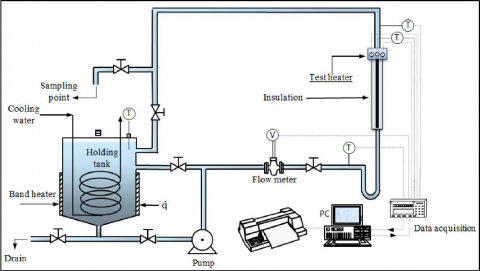Figure 1. A schema of the heat transfer device utilized in this research

The storage tank is made out of stainless steel with an internal diameter of 30 cm and maximum volume of 45 L. The utilized pump was employed with the variability of flow rate between 10 and 100 L/min. The test section consists of a straight vertical pipe made of stainless steel (Type 316) 300 mm long with an internal and external diameter of 23.8 and 25.4 mm, respectively. The schema of the test section is illustrated in Figure 2.

## 2.png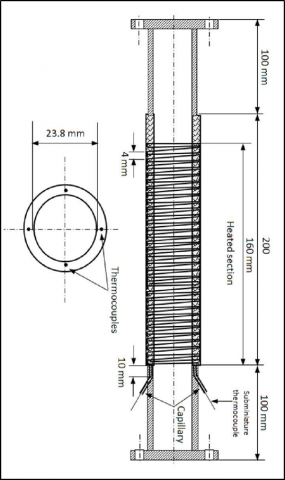Figure 2. A simple schema of the test section

A heating system surrounds the pipe of the test section spirally, and the heat transfer section is fed by a DC electricity source with a maximum power of 1000 W. In order to prevent heat exchange with the environment, the heating section was covered by an insulation of glass wool with a thickness of 20 mm. Four thin thermocouples were installed at sites device onto the pipes and very close to the wall. These thermocouples were utilized for measuring the internal temperature on the wall of the pipe and were of K-Type. These thermocouples were already calibrated based on the thickness of 0.4 mm off the internal surface of the pipe, thus the wall's temperature will fall in relation to the value read by the thermocouples. Considering this drop, the temperature of the wall was read by the Eq(1).

The mean value read by the three thermocouples is utilized for calculating heat transfer coefficient, where the fourth thermocouple is connected to a heat control system in order to stop excessive growth of temperature in the test section. Two other thermocouples were also used in the input and output section of the flow to the test section in order to measure the input and output temperature of the fluid. Thermocouples were calibrated by a thermostat-equipped bath, where the measurement was performed with an accuracy of ±0.01 %. The length of the heating section

was 160 mm and the thermocouples were installed 95 mm off the beginning of the heating section. To measure the velocity, ABBKENT device (2600T) was utilized to measure the volume flow rate.

$T_{w}=T_{t h}-\frac{q^{\prime}}{k / \Delta S}$    (1)

2.4. Heat transfer coefficient measurement

To measure the heat transfer coefficient first, distilled water was poured into the storage tank. Next, it was rotated by a pump in the system complex. After about 50-60 min, the system finds a steady-state. All measurements were performed under steady conditions. During the experiment, the temperature of the output and input fluid to the test section, and the wall's temperature were measured by the in-situ three thermocouples (95 mm off) and the volume flow rate. The flow rate was adjusted by a valve in the reverse flow. The experiment was performed in 16 different rates and for each point the experiment was replicated at least two times. Following the experiment for distilled water, the experiment was also performed for magnesium oxide nanoparticles at five different concentrations and 16 different rates, with two replications for each point. The local heat transfer coefficient was experimentally measure at this site of connection of thermocouples.

Energy balance between the energy introduced into the heat transfer section and the energy absorbed by the fluid of Eqs. (2) and (3) along with the local heat transfer coefficient.

$Q=V . I$        (2)

$Q=m \cdot C_{p}\left(T_{b o u t}-T_{b i n}\right)$     (3)

The difference between the energy obtained from Eqs. (2) and (3) is less than ±2.5 %, where temperature loss to the air was neglected. To experimentally calculate the local heat transfer coefficient, Eq. (4) was utilized, and to calculate the heat flux injected to the test section Eq. (5) and for determine experimental Nusselt number, Eq. (6) was used.

$h(x)=q^{\prime} /\left(T_{w}(x)-T_{b}(x)\right.$     (4)

$q^{\prime}=\frac{Q}{\pi D L}$     (5)

$N u_{x}=h(x) D / k$      (6)

In these equations, $q^{\prime}$ is the heat flux injected to the test section. $T_{w}$ and $T_{b}$ are the mean value of the wall's temperature and the mean value of the temperature of fluid bulk within a distance of $X$ within the direction of horizontal axis from the point where the heat enters the pipe, respectively. $D$ is the pipe's diameter, $L$ is the pipe's length, and $k$ is the thermal conductivity of the nanofluid. The mean temperature of the fluid bulk at the point with a distance of $X$ from the heat entrance section can be obtained by the following equation, considering the constant heat flux and assumption of linearity of the changes in the fluid temperature.

$T_{b(x)}=T_{b i n}+\frac{q^{\prime} S X}{\rho C_{p} u A}$       (7)

where, $T_{b i n}$ is the temperature of the fluid bulk in the input, $C_{p}$ is the heat capacity, $\rho$ is the fluid density, $S$ is the environment, and $A$ is the cross-section area of the pipe of the test section, and $\mathcal{U}$ denotes the mean velocity of the fluid which changes as follows, considering the experimental conditions of Eq. (7), thus Eq. (8) was obtained.

$T_{b(x)}=T_{b i n}+\left(T_{b o u t}-T_{b i n}\right) \times \frac{95}{160}$    (8)

3. Required Correlations Selection

3.1 Nanofluid thermal properties

To determine physical properties contain the heat capacity and density of nanofluids, Xuan and Roetzel  and Pak and Cho  models respectively were used. Viscosity is an important physical property of nanofluids whose reduction results in decreased pumping cost. However, typically with the addition of nanoparticles to the base fluid, the viscosity grows, yet in contrast, the heat conductivity increases there by developing heat. Notwithstanding, there are factors influencing the viscosity of nanofluids including the concentration of nanoparticles, the type, size, shape, and type of the base fluid, not to mention the fact that temperature also affects its value. Considering the previous studies , the principal equations in experimental and analytical models include three major models: Einsteins , Brinkman  and Wang et al . These models have been utilized in the majority of studies in recent years as the equation for determining the viscosity of nanofluids and predicting heat transfer models without considering the aggregate effects.

In determining the viscosity by considering the aggregate effect of particles, the model used by many researchers has been the fractal model [23-29], while in this research, the fractal model was compared with conventional models without considering the aggregate effect.

According to earlier studies , several conventional models have been utilized for calculating the heat conductivity of nanofluids without considering the aggregate effect of particles in most of the studies. Based on studies carried out by previous researchers , at low concentrations, the results obtained are similar to each other, and match with the Maxwell  model.

The conventional methods that consider the aggregate effect in consideration are three major models: Xuan and Li. model , fractal model [32-36] and modified fractal model (fractal and nanolayer) [28,37-40]. The accuracy of these models will also be specified and eventually the one with the greatest accuracy will be chosen. In carrying out the experiment and collecting data, the following conditions were taken into consideration:

a) Constant heat flux of 500 W was applied.

b) Each test of heat transfer for each point was replicated at least two times and for determining the physical properties as well as the DLS test, it was replicated three times.

c) The mean temperature of the nanofluid bulk in the experiments were around 300 K.

d) The parameters measured in the heat transfer device included the volume flow rate of the nanofluid, the fluid temperature in the input and output in the measurement section, and the wall's temperature in the measurement section.

3.2 Heat transfer coefficient correlation for distilled water

The device used and the operational conditions of the heat transfer mechanism developed can be regarded as the basis of the proposed model in this research considering the effect of input entry, three main equations of Hausen , Gnielinski , and Tam and Ghajar Eq. .

By conducting the experiment for pure water and comparing it with the values obtained from the three proposed equations, the equation with the lowest error value was chosen as the basis and the proposed model was selected based on the previous studies [44-45].

4. Results And Discussion

4.1 Prediction of heat transfer coefficient of the nanofluid

The deviation between experimental and calculated is presented using the relative absolute average deviation (RAAD)

$\% R A A D=\frac{100}{N}\left(\sum_{i}^{N}\left|\frac{P_{i}^{\mathrm{exp}}-P_{i}^{c a l c}}{P_{i}^{\mathrm{exp}}}\right|\right)$       (9)

where P represents heat transfer coefficient or Nusselt number and N is the number of experimental data points.

Since the RAAD of Gnilienski model for pure water has been around 5 % in this research and is more than 20 % in other models, thus we based our model on Gnilienski model.

## 3.pngFigure 3. The curve of comparing Nusselt numbers obtained from the models and experimental values for distilled water

Figure 3 presents a comparison of the Nusselt numbers obtained from the models and experimental values for distilled water. Therefore, the model proposed for determining the local heat transfer coefficient of the nanofluid considering the previous studies [44-47] is presented in the following equation:

$N u_{n f x}$ = $(f i / 8)$$\left(\operatorname{Re}_{n f}-1000\right) \operatorname{Pr}_{n f}$ / $1+\delta_{v}^{+} \sqrt{f i} /_{8}\left(\operatorname{Pr}_{n f v}^{2 / 3}-1\right)$ $\left[1+\frac{1}{3}(-)^{2 / 3}\right]\left(\frac{\operatorname{Pr}_{n f}}{\operatorname{Pr}_{w n f}}\right)^{0.11}$(10)

$f i=\left[1.82 \log \left(\mathrm{Re}_{n f}\right)-1.64\right]^{-2}$      (11)

The proposed equation, is modified Gnielinski equation in view of the assumptions considered in the research was carried out by Buongiorno .

4.2 Viscosity

Based on the equations presented for determining the viscosity of data fluids, in the aggregation state, the mean hydrodynamic diameter of MgO nanoparticles in the aggregate states needed per different volume concentrations by DLS device is presented in Table 1.

Table 1. The mean hydrodynamic diameter of MgO nanoparticles in the aggregate state

 Volume fraction percent Hydrodynamic diameter (nm) 0.02 449 0.05 330 0.07 320 0.09 331 0.12 312

## 4.png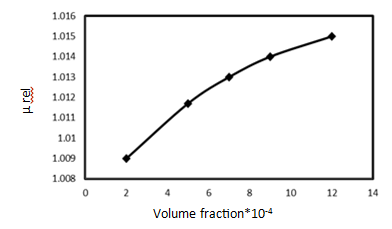Figure 4. The relative viscosity curve of (μrel) in terms of the changes in the volume fraction of the MgO/water nanofluid

## 5.png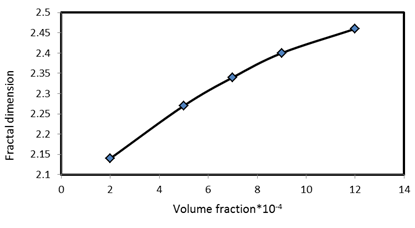Figure 5. The curve for the changes in the fractal dimension of MgO/water nanofluid

Furthermore, the relative viscosity curve ( $\mu_{r e l}$ ) in terms of the changes in the volume fraction of the nanofluid of MgO/water is presented in Figure 4. The curve for the changes in the fractal dimension of MgO/water nanofluid in relation to the variations of volume fraction is presented in Figure 5.

The curve for comparing different models used for determining the viscosity of MgO/water nanofluid in terms of the changes in the volume fraction and experimental values is presented in Fig. 6. It can be observed that for models that do not consider the aggregate effects, Wang et al model presents the best results with the experimental values. However, fractal model, which takes aggregate effect into consideration, presents the best results when compared with all other models.

## 6.png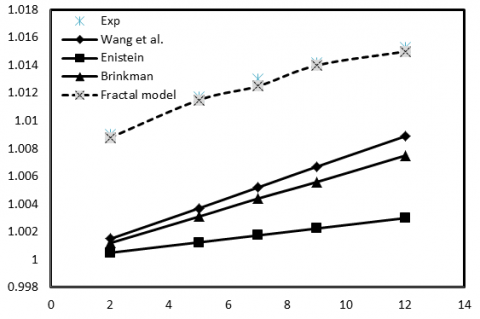Figure 6. The curve for comparing different models used for determining the viscosity of MgO/water nanofluid

4.3. Thermal conductivity

According to Figure 7, comparison between the M.G. model that neglects the aggregate effects with the three models of fractal, Xuan and Li, and fractal and Nano layer, which consider the aggregate effect, reveals that fractal model offers the best responses with the experimental values.

## 7.pngFigure 7. The curve for the comparison of different models used for determining thermal conductivity for MgO/water nanofluid

4.4. Heat transfer results

Figures. 8 and 9 offer the extent of increase in the heat transfer coefficient of the MgO/water nanofluid in comparison with the base fluid of distilled water at different concentrations with different Reynolds number. They indicate that the greatest development was around 12 % for the concentration of 0.12 %, and the mean increase in the heat transfer observed within the entire concentration ranges and all of the Reynolds numbers in relation to the base fluid was 6 %.

## 8.png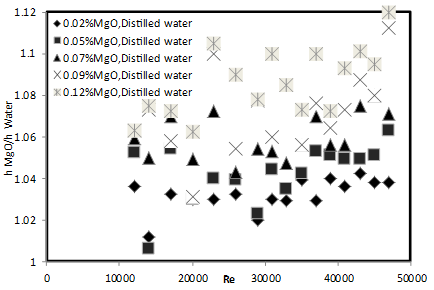Figure 8. The curve for the ratio of heat transfer coefficient of MgO/water nanofluid to distilled water at different concentrations of nanoparticles in terms of the Reynold’s number

## 9.png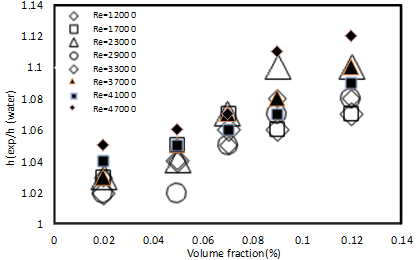Figure 9. The curve for the changes in the ratio of heat transfer coefficient of MgO/water nanofluid to distilled water at different Reynolds number

## 10.png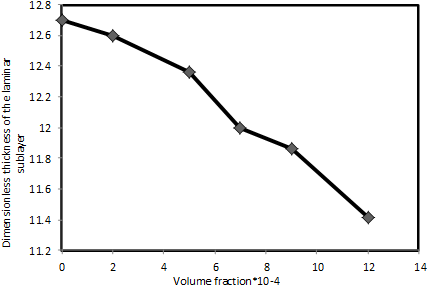Figure 10. The curve for the changes in the dimensionless thickness of the laminar sublayer in terms of the variations in the volume fraction for the MgO/water nanofluid

Based on the proposed model, in Figure. 10, the dimensionless thickness of the laminar sublayer was obtained by having experimental data and considering the physical properties based on the equations without considering the aggregate effect (Wang et al model for viscosity and M.G. model for thermal conductivity) in terms of concentration. It was also observed that with the increase in the concentration, the thickness of the dimensionless sublayer declines and heat transfer also grows because of the increase in Brownian diffusion that can be explained mainly with a reduction of viscosity within consequent thinning of the laminar sub-layer. The obtained results were also similar to Buongiorno. 

Figure. 11 indicates the dimensionless thickness of the laminar sublayer with experimental data and considering the physical properties based on the questions considering the aggregate effect (fractal model for viscosity and thermal conductivity) in terms of the concentration. It was observed that as the concentration increases, dimensionless layer thickness diminishes, where heat transfer also increases, as with the previous state.

Figure. 12 compares the dimensionless thickness of the laminar sublayer in the aggregate and particle states. It can be seen that its value is lower in the particle state, suggesting that aggregation of particles can result in reduced heat transfer. In other words, the Brownian diffusion is lower in the aggregate state than in particle state (no aggregation), leading to diminished the average nanoparticle

## 11.png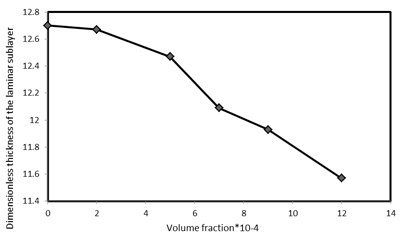Figure 11. The curve for the changes in the dimensionless thickness of the laminar sublayer in terms of the variations in the volume fraction for the MgO/water nanofluid considering the aggregate effect

## 12.pngFigure 12. The curve for comparing the changes in the dimensionless thickness of the laminar sublayer

For instance, in Figures. 13 and 14, the changes in the Nusselt number in relation to the Reynolds number were compared at concentrations of 0.12 % of the MgO nanoparticle in the model state with and without considering the aggregate effect, Gnileinski model, and experimental values. In addition, in Figure. 15 the curve for changes in the convection heat transfer coefficient in relation with Reynolds number at concentrations of 0.12 % of MgO nanoparticle obtained from the models that neglect the aggregate effect, those that consider the aggregate effect, Gnileinski model, and experimental values were compared.

## 13.pngFigure 13. The curve for the changes in the Nusselt number in relation to Reynolds number for the nanofluid of MgO/water with volume concentration of 12×10-4

## 14.png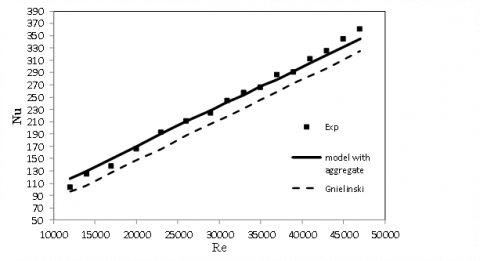Figure 14. The curve for the changes in the Nusselt number in relation to Reynolds number for the nanofluid of MgO/water with volume concentration of 12×10-4 considering the aggregate effect

## 15.png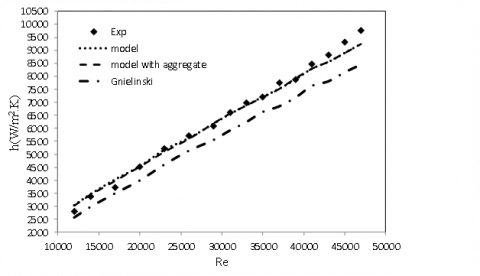Figure 15. The curve for the changes in the convection heat transfer coefficient in relation to Reynolds number for MgO/water nanofluid with volume concentration of 0.12 %

Note that in the models that neglect the aggregate effect, the conductivity of nanofluid was obtained by M.G. model and the viscosity was calculated by Wang et al model. To calculate the dimensionless thickness of the laminar sublayer, the mean diameter of nanoparticles in the particle state was used. In the models that consider the aggregate effect, to determine the nanofluids conductivity and viscosity, the fractal model was used. To calculate the dimensionless thickness of the laminar sublayer, the mean diameter of the aggregates in the bulking state was used. Table 2 shows the comparison of the RAAD of the models in relation to the experimental value within the range of applied Reynolds number along with the utilized concentrations. It was observed that with the increase in concentration, the RAAD in Gnileinski model increases as well. In addition, the RAAD of models in the state of aggregation and lack of aggregation is almost similar, where the RAAD of Gnileinski equation is about three times that of other models.

Table 2. Comparing the error of models in terms of concentration for the MgO nanofluid within the range of applied Reynolds number

 Volume fraction 2×10-4 5×10-4 7×10-4 9×10-4 12×10-4 % RAAD without aggregate 2.5 2.6 2.4 3.26 2.8 % RAAD with aggregate 2.58 2.48 2.26 3.15 2.7 % RAAD Gnielinski 5.7 6.5 7.96 8.4 10.4
5. Conclusions

The following conclusion has been drawn from the present study:

1. The addition of MgO nanoparticles to the base fluid of water even at low concentrations (0.02-0.12 %) within the Reynolds number range of 11,000-49,000 results in increased heat transfer coefficient up to 12 and 6 % on average.

2. In calculating the conductivity and viscosity of the MgO/water nanofluid, considering the aggregate effects, fractal models present the best responses in relation to the experimental values.

3. In calculating the conductivity and viscosity of the MgO/water nanofluid, without considering the aggregate effects, Wang et al model present the best responses in relation to the experimental values.

4. The proposed model had a RAAD of about 2.5 % in relation to the experimental values in both the aggregation and lack of aggregation states, and the aggregate effect is negligible in this concentration range.

5. The RAAD of Gnileinski model is 2-4 times and 3 times on average greater than the RAAD of the proposed model within the experimented concentration range and Reynolds number range.

6. The thickness of the dimensionless laminar sublayer, considering the aggregate effects, is greater than the thickness of the dimensionless laminar sublayer without considering the aggregate effect due to reduction in the Brownian diffusion. Therefore, it is predicted that aggregation can result in reduced heat transfer in nanofluids.

Acknowledgment

The authors would like to express their appreciation to the Petroleum University of Technology (PUT) for providing financial support for this study.

Nomenclature
 $A$ tube cross section area, m2 $C_{p}$ specific heat capacity, J.kg-1.K-1 $D$ tube diameter, m $f i$ friction factor $h$ heat transfer coefficient, W.m-2.K-1 $k$ thermal conductivity, W.m-1.K-1 $L$ length of tube, m $m^{\bullet}$ mass flow rate, kg.s-1 $N_{n}$ Nusselt number Pr Prandtl number $q^{\bullet}$ heat flux, W.m-2 $Q$ injected energy,W Re Reynolds number $S$ tube perimeter, m $T$ temperature, K $\mathcal{U}$ mean velocity of fluid, m.s-1 $X$ axial distance from the entrance,m Greek Symbols $\delta_{v}^{+}$ dimensionless thickness of the laminar sublayer $\Delta S$ thickness of tube, m $\mu$ viscosity, kg.m-1.s-1 $\rho$ density, kg.m-3 Subscripts $b$ bulk $n f$ nanofluid $r e l$ relative $t h$ thermocouple $v$ laminar sublayer $\mathcal{W}$ wall $x$ local Abbreviations RAAD Relative Absolute Average Deviation DLS Dynamic Light Scattering MG Maxwell-Granett
References

 Choi U.S., Eastman J.A. (1995). Enhancing thermal conductivity of fluids with nanoparticles, ASME FED, Vol. 231, pp. 99-105.

 Pak B.C., Cho Y.I. (1998). Hydrodynamic and heat transfer study of dispersed fluids with submicron metallic oxide particles, Experimental Heat Transfer, Vol. 11, No. 2, pp. 151-170. DOI: 10.1080/08916159808946559

 Xuan Y., Li Q. (2004). Flow and heat transfer performances of nanofluids inside small hydraulic diameter flat tube, Journal of Engineering Thermophysics, Vol. 25, pp. 305-307.

 He Y., Jin Y., Chen H., Ding Y., Cang D., Lu H. (2007). Heat transfer and flow behaviour of aqueous suspensions of TiO2 nanoparticles (nanofluids) flowing upward through a vertical pipe, International Journal of Heat and Mass Transfer, Vol. 50, No. 11-12, pp. 2272-2281. DOI: 10.1016/j.ijheatmasstransfer.2006.10.024

 Duangthongsuk W., Wongwises S. (2009). Heat transfer enhancement and pressure drop characteristics of TiO2–water nanofluid in a double-tube counter flow heat exchanger, International Journal of Heat and Mass Transfer, Vol. 52, No. 7-8, pp. 2059-2067. DOI: 10.1016/j.ijheatmasstransfer.2008.10.023

 Sajadi A., Kazemi M. (2011). Investigation of turbulent convective heat transfer and pressure drop of TiO2/water nanofluid in circular tube, International Communications in Heat and Mass Transfer, Vol. 38, No. 10, pp. 1474-1478. DOI: 10.1016/j.icheatmasstransfer.2011.07.007

 Sundar L.S., Naik M., Sharma K., Singh M., Reddy T.S. (2012). Experimental investigation of forced convection heat transfer and friction factor in a tube with Fe3O4 magnetic nanofluid, Experimental Thermal and Fluid Science, Vol. 37, pp. 65-71. DOI: 10.1016/j.expthermflusci.2011.10.004

 Kanna P.R., Taler J., Anbumalar V., Kumar A.V., Pushparaj A., Christopher D.S. (2014). Conjugate heat transfer from sudden expansion using nanofluid, Numerical Heat Transfer, Part A: Applications, Vol. 67, No. 1, pp. 75-99. DOI: 10.1080/10407782.2014.915685

 Sivakumar A., Alagumurthi N., Senthilvelan T. (2015). Experimental and numerical investigation of forced convective heat transfer coefficient in nanofluids of Al2O3/water and CuO/EG in a serpentine shaped microchannel heat sink, International Journal of Heat and Technology, Vol. 33, No. 1, pp. 155-160.  DOI: 10.18280/ijht.330121

 Guzei D.V., Minakov A.V., Rudyak V.Y. (2016). Investigation of heat transfer of nanofluids in turbulent flow in a cylindrical channel, Fluid Dynamics,Vol. 51, No. 2, pp. 189-199. DOI: 10.1134/s0015462816020071

 Ahrara A.J., Djavareshkianb M.H., Ataiyanc M. (2017). Numerical simulation of Cu-water nanofluid magneto-hydro-dynamics and heat transfer in a cavity containing a circular cylinder of different size and positions, International Journal of Heat and Technology, Vol. 35, No. 2, pp. 403-415. DOI: 10.18280/ijht.350225

 Duangthongsuk W., Wongwises S. (2008). Effect of thermophysical properties models on the predicting of the convective heat transfer coefficient for low concentration nanofluid, International Communications in Heat and Mass Transfer, Vol. 35, No. 10, pp. 1320-1326. DOI: 10.1016/j.icheatmasstransfer.2008.07.015

 Motevasel M., Soleimanynazar A., Jamialahmadi M. (2014). Comparing mathematical models to calculate the thermal conductivity of nanofluids, American Journal of Oil and Chemical Technology, Vol. 2, No. 11, pp. 359-369.

 Xuan Y., Li Q. (2000). Heat transfer enhancement of nanofluids, International Journal of Heat and Fluid Flow, Vol. 21, No. 1, pp. 58-64. DOI: 10.1016/S0142-727X(99)00067-3

 Wen D., Ding Y. (2005). Formulation of nanofluids for natural convective heat transfer applications, International Journal of Heat and Fluid Flow, Vol.26, No. 6, pp. 855-864. DOI: 10.1016/j.ijheatfluidflow.2005.10.005

 Meriläinen A., Seppälä A., Saari K., Seitsonen J., Ruokolainen J., Puisto S., Ala-Nissila T. (2013). Influence of particle size and shape on turbulent heat transfer characteristics and pressure losses in water-based nanofluids, International Journal of Heat and Mass Transfer, Vol. 61, pp. 439-448. DOI: 10.1016/j.ijheatmasstransfer.2013.02.032

 Xie H., Li Y., Yu W. (2010). Intriguingly high convective heat transfer enhancement of nanofluid coolants in laminar flows, Physics Letters A, Vol. 374, No. 25, pp. 2566-2568. DOI: 10.1016/j.physleta.2010.04.026

 Xie H., Yu W., Chen W. (2010). MgO nanofluids: higher thermal conductivity and lower viscosity among ethylene glycol-based nanofluids containing oxide nanoparticles, Journal of Experimental Nanoscience, Vol. 5, No. 5, pp. 463-472. DOI: 10.1080/17458081003628949

 Xuan Y., Roetzel W. (2000). Conceptions for heat transfer correlation of nanofluids, International Journal of Heat and Mass Transfer, Vol. 43, No. 19, pp. 3701-3707. DOI: 10.1016/s0017-9310(99)00369-5

 Einstein A. (1956). Investigation on theory of Brownian motion, Dover, New York, pp. 19-22.

 Brinkman H.C. (1952). The viscosity of concentrated suspensions and solutions, The Journal of Chemical Physics, Vol. 20, No. 4, pp. 571-571. DOI: 10.1063/1.1700493

 Wang X., Xu X., Choi S.U. (1999). Thermal conductivity of nanoparticle - Fluid mixture, Journal of Thermophysics and Heat Transfer, Vol, 13, No. 4, pp. 474-480. DOI: 10.2514/2.6486

 Wang X., Xu X., Choi S.U. (1999). Thermal conductivity of nanoparticle - Fluid mixture, Journal of Thermophysics and Heat Transfer, Vol. 13, No. 4, pp. 474-480. DOI: 10.2514/2.6486

 Gharagozloo P.E., Goodson K.E. (2011). Temperature-dependent aggregation and diffusion in nanofluids, International Journal of Heat and Mass Transfer, Vol. 54, No. 4, pp. 797-806. DOI: 10.1016/j.ijheatmasstransfer.2010.06.058

 Wang T., Ni M., Luo Z., Shou C., Cen K. (2012). Viscosity and aggregation structure of nanocolloidal dispersions, Chinese Science Bulletin, Vol. 57, No. 27, pp. 3644-3651. DOI: 10.1007/s11434-012-5150-y

 G., & Srivastava S. (2012). Effect of aggregation on thermal conductivity and viscosity of nanofluids, Applied Nanoscience, Vol. 2, No. 3, pp. 325-331. DOI: 10.1007/s13204-012-0082-z

 Kok C.M., Rudin A. (1981). Relationship between hydrodynamic radius and the radius of gyration of a polymer in solution, Macromolecular Rapid Communication, Vol. 2, pp. 655-659. DOI: 10.1002/marc.1981.030021102

 Rooij R.D., Potanin A.A., Ende D.V., Mellema J. (1993). Steady shear viscosity of weakly aggregating polystyrene latex dispersions, The Journal of Chemical Physics, Vol. 99, No. 11, pp. 9213-9223. DOI: 10.1063/1.465537

 Chýlek P., Srivastava V. (1983). Dielectric constant of a composite inhomogeneous medium, Physical Review B, Vol, 27, No. 8, pp. 5098-5106. DOI: 10.1103/physrevb.27.5098

 Maxwell Granett J.C. (1904) Colours in metal glasses and in metallic films, Philosophical Transactions of the Royal Society A, Vol. 203, pp. 385-420. DOI: 10.1098/rsta.1904.0024

 Xuan Y., Li Q., Hu W. (2003). Aggregation structure and thermal conductivity of nanofluids, AIChE Journal, Vol. 49, No. 4, pp. 1038-1043. DOI: 10.1002/aic.690490420

 Wang B., Zhou L., Peng X. (2003). A fractal model for predicting the effective thermal conductivity of liquid with suspension of nanoparticles, International Journal of Heat and Mass Transfer, Vol. 46, No. 14, pp. 2665-2672. DOI: 10.1016/s0017-9310(03)00016-4

 Thomas J.C. (1987). The determination of log normal particle size distributions by dynamic light scattering, Journal of Colloid and Interface Science, Vol. 117, NO. 1, pp. 187-192. DOI: 10.1016/0021-9797(87)90182-2

 Bruggeman D.A.G. (1935). Berechnung verschiedener physikalischer konstanten von heterogenen substanzen, I. dielektrizitatskonstanten und leitfahigkeiten der mischkorperausIsotropen substanzen, Annalen der Physik. Leipzig, Vol. 24, pp. 639-679.

 Hui P.M., Stroud D. (1986). Complex dielectric response of metal-particle clusters, Physical Review B, Vol. 33, No. 4, pp. 2163-2169. DOI: 10.1103/physrevb.33.2163

 Hui P.M., Stroud D. (1994). Effective linear and nonlinear response of fractal clusters, Physical Review B, Vol. 49, No. 17, pp. 11729-11735. DOI: 10.1103/physrevb.49.11729

 Yu W., Choi S. (2003). The role of interfacial layers in the enhanced thermal conductivity of nanofluids: A renovated maxwell model, Journal of Nanoparticle Research, Vol. 5, No. 1-2, pp. 167-171. DOI: 10.1023/a:1024438603801

 Xie H., Fujii M., Zhang, X. (2005). Effect of interfacial nanolayer on the effective thermal conductivity of nanoparticle-fluid mixture, International Journal of Heat and Mass Transfer, Vol. 48, No. 14, pp. 2926-2932. DOI: 10.1016/j.ijheatmasstransfer.2004.10.040

 Dwornick B.L., Jeyashekar N.S., Johnson J.E., Schall J.D., Comfort A.S., Zou Q., Dusenbury J.S., Thrush S.J., Powers C.C., Hutzler S.A., Frame E.A. (2012). Application of dynamic light scattering to characterize nanoparticle agglomeration in alumina nanofluids and its effect on thermal conductivity, U.S. Army TARDEC Report, 23101, 11 Jul. 2012. http://www.dtic.mil/get-tr-doc/pdf?AD=ADA566654

 Özerinç S., Kakaç S., Yazıcıoğlu A.G. (2009). Enhanced thermal conductivity of nanofluids: A state-of-the-art review, Microfluidics and Nanofluidics, Vol. 8, No. 2, pp. 145-170. DOI: 10.1007/s10404-009-0524-4

 Hausen H. (1959). New equation for heat transfer in free or force flow, Allg. Warmetchn, Vol. 9, No. 4-5, pp. 75-79.

 Gnielinski V. (1986). Wa''rmeu''bergang in Rohren, Vdi-Wa'rmeatlas, Fifth Ed., VDI-Verlag, Du" sseldorf

 Ghajar A.J., Tam L. (1994). Heat transfer measurements and correlations in the transition region for a circular tube with three different inlet configurations, Experimental Thermal and Fluid Science, Vol. 8, No. 1, pp. 79-90. DOI: 10.1016/0894-1777(94)90075-2

 Filonenko G.K. (1954). Hydraulic resistance in pipes, Teploenergetika, Vol. 1, pp. 40-44.

 Buongiorno J. (2006). Convective transport in nanofluids, Journal of Heat Transfer, Vol. 128, No. 3, pp. 240-250. DOI: 10.1115/1.2150834

 Prandtl L. (1994). Füehrer Durch die ströemungslenhre, Vieweg, Braunschweig, pp. 359-360.

 McNab G., Meisen A. (1973). Thermophoresis in liquids, Journal of Colloid and Interface Science, Vol. 44, No. 2, pp. 339-346. DOI: 10.1016/0021-9797(73)90225-7